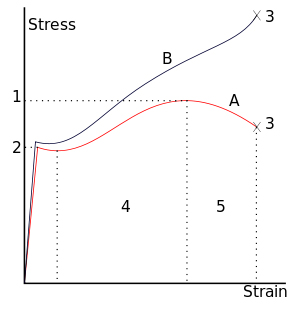# What is the Difference between Starin and Stress?

### What is Strain?

To make it easier for you to understand, let’s merge the definition of stress with strain.
When an object is subjected to an external load, the internal resistance which is built up with the object itself is not enough to withstand the external load results into deformation of the object. This alteration or deformation of the object is called strain.

The formula for strain is given as follows.

• Strain- Change in length/Original length
• Strain has no unit.### What is stress?

When an object is subjected to an external force, the object tends to build up internal resistance within itself material. This resistance is termed as “stress”.

### Stress can be classified into four types.

• Compressive stress
• Tensile stress
• Bending stress
• Shear stress

Stress- Load/Area = W/A = N/mm2
Where, N = Newton